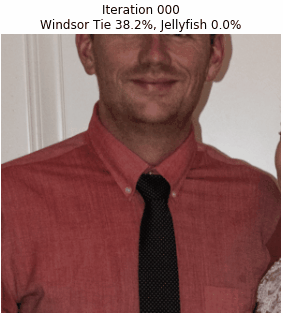# Tricking Deep Learning

Deep neural networks have had remarkable success with many tasks including image recognition. Read this overview regarding deep learning trickery, and why you should be cognizant.

Since Neural Networks and Deep Learning are becoming such popular themes we show a few areas where they have substantial room for improvement. Based on the work of Anh Nguyen et al we show how easy it is to manipulate some of these networks.Here we make very subtle changes to the image to have the network go from thinking it is a picture of a tie to thinking it is a jellyfish.

### How

Basically we use the same basic process for teaching the network to recognize the image. Instead of changing the weights to optimize the outcome, we change the input image.

To visually represent this we will use an idea of a computational graph. Basically this graph shows a flow chart of how the final result is computed. Since Deep Neural Networks are so named because they have so many layers these are not always easy to draw. The image below shows just part of a standard image classification graph. We can look at smaller pieces to understand them better.The bottom few layers of the Inception V3 network used in this test

### Standard Training

Looking at a small section of the network we can describe what the training process does in more detail. Each node represents either an operation or a value and the arrows show how information flows through the system. Google’s Deep Learning framework, TensorFlow, is named after exactly this idea of tensors (images, matrices, words, vectors, …) flowing through such systems. The important aspect for training is breaking the network into two components. The first is the output, in this case softmax. This is used to create the loss function (not shown) which is the way of scoring how well the network is accomplishing the desired task. The second are the variables, in this case softmax/weights and softmax/biases. The variables are the portions which can be changed and updated to improve the final result.A small piece of the final stages of the Inception network. The dashed blue line represents a large number of layers which are not shown to keep the figure from becoming monstrous. The red arrows show the values which are being optimized in the network and the green value shows the part which is being ‘optimized’. In this case the softmax is compared with the trained labels to minimize the classification error.

### From Inception to Deception

Here we change the way the training works to make changes or updates to the input image instead of the weights and biases. We also modify the loss function and output so it tries to increase the jellyfish-ness. The network is then run through many iterations with each iteration causing a small change to the input image.The same piece of network but instead of using the softmax, we compare the softmax to the one-hot jellyfish vector and try to increase that value. Furthermore instead of adjusting the weights and biases, the changes are made to original input image.